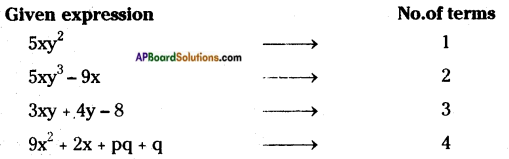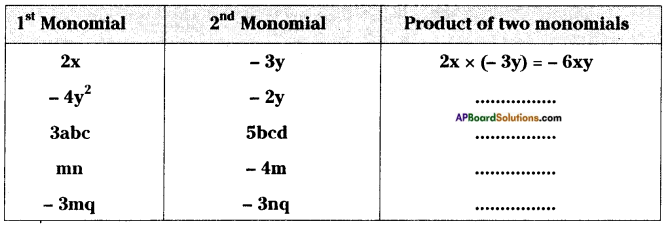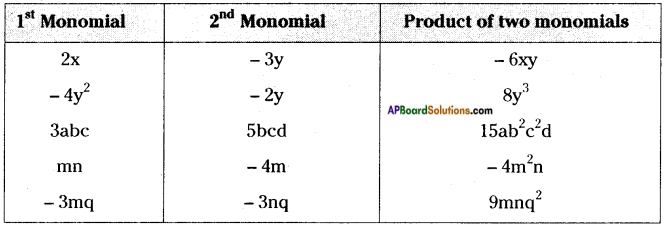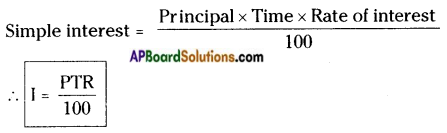# HSSlive: Plus One & Plus Two Notes & Solutions for Kerala State Board

## AP Board Class 8 Maths Chapter 11 Algebraic Expressions InText Questions Textbook Solutions PDF: Download Andhra Pradesh Board STD 8th Maths Chapter 11 Algebraic Expressions InText Questions Book AnswersAP Board Class 8 Maths Chapter 11 Algebraic Expressions InText Questions Textbook Solutions PDF: Download Andhra Pradesh Board STD 8th Maths Chapter 11 Algebraic Expressions InText Questions Book Answers

## Andhra Pradesh State Board Class 8th Maths Chapter 11 Algebraic Expressions InText Questions Books Solutions

 Board AP Board Materials Textbook Solutions/Guide Format DOC/PDF Class 8th Subject Maths Chapters Maths Chapter 11 Algebraic Expressions InText Questions Provider Hsslive

2. Click on the Andhra Pradesh Board Class 8th Maths Chapter 11 Algebraic Expressions InText Questions Answers.
3. Look for your Andhra Pradesh Board STD 8th Maths Chapter 11 Algebraic Expressions InText Questions Textbooks PDF.
4. Now download or read the Andhra Pradesh Board Class 8th Maths Chapter 11 Algebraic Expressions InText Questions Textbook Solutions for PDF Free.

## AP Board Class 8th Maths Chapter 11 Algebraic Expressions InText Questions Textbooks Solutions with Answer PDF Download

Find below the list of all AP Board Class 8th Maths Chapter 11 Algebraic Expressions InText Questions Textbook Solutions for PDF’s for you to download and prepare for the upcoming exams:

Do this

Question 1.
Find the number of terms in following algebraic expressions.
5xy2, 5xy3 – 9x, 3xy + 4y – 8, 9x2 + 2x + pq + q. [Page No. 248]Question 2.
Take different values for x and find values of 3x + 5. [Page No. 248]
If x = 1 then 3x + 5 = 3(1) + 5 = 3 + 5 = 8
If x = 2 then 3x + 5 = 3(2) + 5 = 6 + 5 = 11
If x = 3 then 3x + 5 = 3(3) + 5 = 9 + 5 = 14

Question 3.
Find the like terms in the following: ax2y, 2x, 5y2, -9x2, -6x, 7xy, 18y2. [Pg. No. 249]
Like terms are (2x, – 6x) (5y2, 18y2).

Question 4.
Write 3 like terms for 5pq2. [Pg. No. 249]
Like terms of 5pq2 are – 3pq2, pq2, pq22etc.,

Question 5.
If A = 2y2 + 3x – x2, B = 3x2 – y2 and C = 5x2 – 3xy then find [Pg. No. 250]
(i) A + B (ii) A – B (iii) B + C (iv) B – C (v) A + B + C (vi) A + B – C
A = 2y2 + 3x – x2, B = 3x2 – y2, C = 5x2 – 3xy
i) A + B = (2y2 + 3x – x2) + (3x2 – y2)
= (2y2 – y2) + 3x + (3x2 – x2)
∴ A + B = y2 + 3x + 2x2 = 2x2 + 3x + y2

ii) A – B = (2y2 + 3x – x2) – (3x2 – y2)
= 2y2 + 3x – x2 – 3x2 + y2
∴ A – B = 3y2 + 3x – 4x2

iii) B + C = (3x2 – y2) + (5x2 – 3xy)
= 3x2 + 5x2 – y2 – 3xy
∴ B + C = 8x2 – y2 – 3xy

iv) B – C = (3x2 – y2) – (5x2 – 3xy)
= 3x2 – y2 – 5x2 + 3xy
∴ B – C = – 2x2 – y2 + 3xy

v) A + B + C = A + (B + C)
= (2y2 + 3x – x2) + (8x2 – y2 – 3xy)
= (8x2 – x2) + (2y2 – y2) + 3x – 3xy
∴ A + B + C = 7x2 + y2 + 3x – 3xy

vi) A + B – C = A + (B – C)
= (2y2 + 3x – x2) + (-2x2 – y2 + 3xy)
= (2y2 – y2) + (-x2 – 2x2) + 3x + 3xy :
∴ A + B – C = y2 – 3x2 + 3x + 3xy

Question 6.
Complete the table: [Page No. 253]Question 7.
Check whether you always get a monomial when two monomials are multiplied. [Page No. 253]
Yes, the product of two monomials is always a monomial.
Ex: 2xy × 5y = 10xy is a monomial.

Question 8.
Product of two monomials is a monomial? Check. [Pg. No. 253]
Yes, the product of two monomials is a monomial.
∵ 2x × y = 2xy

Question 9.
Find the product: (i) 3x(4ax + 8by) (ii) 4a2b(a – 3b) (iii) (p + Sq2) pq (iv) (m3 + n3) 5mn2 [Pg. No. 255]
i) 3x (4ax + 8by) = 3x × 4ax + 3x × 8by
= 12ax2 + 24bxy

ii) 4a2b (a – 3b) = 4a2b × a – 4a2b × 3b
= 4a3b – 12a2b2

iii) (p + 3q2) pq = p × pq + 3q2 × pq
= p2q + 3pq3

iv) (m3 + n3) 5mn2 = m3 × 5mn2 + n3 × 5mn2
= 5m4n2 + 5mn5

Question 10.
Find the number of maximum terms in the product of a monomial and a binomial? [Pg. No. 255]
The no.of terms in the product of a monomial and a binomial are two (2).

Question 11.
Find the product: [Pg. No. 257]
(i) (a – b) (2a + 4b)
(ii) (3x + 2y) (3y – 4x)
(iii) (2m – l)(2l – m)
(iv) (k + 3m)(3m – k)
i) (a – b) (2a + 4b) = a(2a + 4b) – b(2a + 4b)
= (a × 2a + a × 4b) – (b × 2a + b × 4b)
= 2a2 + 4ab – (2ab + 4b2)
= 2a2 + 4ab – 2ab – 4b2
= 2a2 + 2ab – 4b2

ii) (3x + 2y) (3y – 4x) = 3x(3y – 4x) + 2y(3y – 4x)
= 9xy – 12x2 + 6y2 – 8xy
= xy – 12x2 + 6y2

iii) (2m – l) (2l – m) = 2m(2l – m) – l(2l – m)
= 2m × 2l – 2m × m – l × 2l + l × m
= 4lm – 2m2 – 2l2 + lm
= 5lm – 2m2 – 2l2

iv) (k + 3m) (3m – k) = k(3m – k) + 3m(3m – k)
= k × 3m – k × k + 3m × 3m – 3m × k
= 3km – k2 + 9m2 – 3km
= 9m2 – k2

Question 12.
How many number of terms will be there in the product df two binomials? [Page No. 257]
No. of terms in the product of two binomials are 4.
Ex: (a + b) (c + d) = ac + ad + be + bd

Question 13.
Verify the following are identities by taking a, b, c as positive integers. [Pg. No. 260]
(i) (a – b)2 = a2 – 2ab + b2
(ii) (a + b) (a – b) = a2 – b2
(iii) (a + b + c)2 = a2 + b2 + c2 + 2ab + 2bc + 2ca
i) (a – b)2 = a2 – 2ab + b2
a = 3, b = 1
⇒ (3 – 1)2 = (3)2 – 2 × 3 × 1 + 12
⇒ (2)2 = 9 – 6 + 1
⇒ 4 = 4
∴ (i) is an identity,

ii) (a + b) (a – b) = a2 – b2
a = 2, b = 1
⇒ (2 + 1) (2 – 1) = (2)2 – (1)2
⇒ 3 × 1 = 4 – 1
⇒ 3 = 3
∴ (ii) is an identity.

iii) (a + b + c)2 = a2 + b2 + c2 + 2ab + 2bc + 2ca
a = 1, b = 2, c = 0
⇒ (1 + 2 + 0)2 = 12 + 22 + 02 + 2 × 1 × 2 + 2 × 2 × 0 + 2 × 0 × 1
⇒ (3)2 = 1 + 4 + 0 + 4 + 0 + 0
⇒ 9 = 1 + 4 + 4
⇒ 9 = 9
∴ (iii) is an identity.

Question 14.
Now take x = 2, a = 1 and b = 3, verify the identity (x + a) (x + b) s x + (a + b)x + ab. [Pg. No. 260]
i) What do you observe? Is LHS = RHS?
ii) Take different values for x, a and b for verification of the above identity.
iii) Is it always LHS = RHS for all values of a and b?
i) (x + a) (x + b) = x2 + (a + b)x + ab
x = 2, a = 1, b = 3 then
⇒ (2 + 1) (2 + 3) = 22 + (1 + 3)2 + 1 × 3
⇒ 3 × 5 = 4 + 4x2 + 3
⇒ 15 = 4 + 8 + 3 ⇒ 15 = 15
∴ LHS = RHS

ii) x = 0, a = 1, b = 2 then
⇒ (0 + 1) (0 + 2) = 02 + (1 + 2) 0 + 1 × 2
⇒ 1 × 2 = 0 + 0 + 2
⇒ 2 = 2
∴ LHS = RHS for different values of x, a, b.

iii) LHS = RHS for all the values of a, b.

Question 15.
Consider (x + p) (x + q) = x + (p + q)x + pq.
(i) Put q instead of ‘p’ what do you observe?
(ii) Put p instead of ‘q’ what do you observe?
(iii) What identities you observed in your results? [Pg. No. 261]
i) (x + p) (x + q) = x2 + (p + q)x + pq …… (1)
Substitute q instead of p in (1).
⇒ (x + q) (x + q) = x2 + (q + q)x + q × q
⇒ (x + q)2 = x2 + 2qx + q2

ii) Substitute ‘p’ instead of q in (1).
⇒ (x + p) (x + p) = x2 + (p + p)x + p × p
⇒ (x + p) = x2 + 2px + p2

iii) ∴ I observe the following identities.
(x + q)2 = x2 + 2qx + q2
(x + p)2 = x2 + 2px + p2

Question 16.
Find: (i) (5m + 7n)2
(ii) (6kl + 7mn)2
(iii) (5a2 + 6b2)2
(iv) 3022
(v) 8072
(vi) 7042
(vii) Verify the identity: (a – b)2 = a2 – 2ab + b2, where a = 3m and b = 5n. [Pg. No. 261]
i) (5m + 7n)2 is in the form of (a + b)2.
(a + b)2 = a2 + 2ab + b2 [a = 5m, b = 7n]
(5m + 7n)2 = (5m)2 + 2 × 5m × 7n + (7n)2
= (5m × 5m) + 70 mn + 7n × 7n
= 25m2 + 70mn + 49n2

ii) (6kl + 7mn)2
We know that (a + b)2 = a2 + 2ab + b2
∴ (6kl + 7mn)2 = (6kl)2 + 2 × 6kl × 7mn + (7mn)2
= 36 k2l2 + 84 klmn + 49 m2n2

iii) (5a2 + 6b2)2
a = 5a2, b = 6b2
(5a2 + 6b2)2 = (5a2)2 + 2 × 5a2 × 6b2 + (6b2)2
= (5a2 × 5a2) + 60a2b2 + (6b2 × 6b2)
= 25a4 + 60a2b2 + 36b4

iv) (302)2 = (300 + 2)2
a = 300, b = 2
∴ (300 + 2)2 = (300)2 + 2 × 300 × 2 + (2)2
= (300 × 300) + 1200 + (2 × 2)
= 90,000 + 1200 + 4
= 91,204

v) (807)2 = (800 + 7)2
a = 800, b = 7
∴ (800 + 7)2 = (800)2 + 2 × 800 × 7 + (7)2
= (800 × 800) + 11,200 + (7 × 7)
= 6,40,000 + 11,200 + 49
= 6,51,249

vi) (704)2 = (700 + 4)2
a = 700, b = 4
∴ (700 + 4)2 = (700)2 + 2 × 700 × 4 + 42
= (700 × 700) + 5600 +(4 × 4)
= 4,90,000 + 5600 + 16
= 4,95,616

vii) (a – b)2 = a2 – 2ab + b2 …… (1)
Substitute a = 3m, b = 5n in (1).
LHS = (3m – 5n)2 = (3m)2 – 2 × 3m × 5n + (5n)2
= 9m2 – 30mn + 25n2
RHS = (3m)2 – 2 × 3m × 5n + (5n)2
= 9m2 – 30mn + 25n2
∴ LHS = RHS

Question 17.
Find:
(i)(9m – 2n)2
(ii) (6pq – 7rs)2
(iii) (5x2 – 6y2)2
(iv) 2922
(v) 8972
(vi) 7942 [Pg. No. 262]
i) (9m – 2n)2 is in the form of (a – b)2.
(a – b)2 = a2 – 2ab + b2
(9m – 2n)2 = (9m)2 – 2 × 9m × 2n + (2n)2
= (9m × 9m) – 36mn + (2n × 2n)
= 81m2 – 36mn + 4n2

ii) (6pq – 7rs)2
a = 6pq, b = 7rs
(6pq – 7rs)2 = (6pq)2 – 2 × 6pq × 7rs + (7rs)2
= (6pq × 6pq) – 84pqrs + (7rs × 7rs)
= 36p2q2 – 84pqrs + 49r2s2

iii) (5x2 – 6y2)2 = (5x2)2 – 2 × 5x2 × 6y2 + (6y2)2
= (5x2 × 5x2) – 60x2y2 + (6y2 × 6y2)
= 25x4 – 60x2y2 + 36y4

iv) (292)2 = (300 – 8)2
a = 300, b = 8
∴ (300 – 8)2 = (300)2 – 2 × 300 × 8 + (8)2 = (300 × 300) – 4800 + (8 × 8)
= 90,000 – 4800 + 64
= 90,064 – 4800
= 85,264

v) (897)2 = (900 – 3)2
= (900)2 – 2 × 900 × 3 + (3)2
= 8,10,000 – 5400 + 9
= 8,10,009 – 5400
= 8,04,609

vi) (794)2 = (800 – 6)2
= (800)2 – 2 × 800 × 6 + (6)2
= 6,40,000 – 9600 + 36
= 6,40,036 – 9600
= 6,30,436

Question 18.
Find:
(i) (6m + 7n) (6m – 7n)
(ii) (5a + 10b) (5a – 10b)
(iii) (3x2 + 4y2) (3x2 – 4y2)
(iv) 106 × 94
(v) 592 × 608
(vi) 922 – 82
(vi) 9842 – 162 [Pg. No. 262]
i) (6m + 7n) (6m -,7n) is in the form of (a + b) (a – b). (a + b) (a – b) = a2 – b2,
here a = 6m, b = 7n
(6m + 7n) (6m – 7n) = (6m)2 – (7n)2
= 6m × 6m – 7n × 7n
= 36m2 – 49n2

ii) (5a + 10b) (5a – 10b) = (5a)2 – (10b)2 [∵ (a + b) (a – b) = a2 – b2]
= 5a × 5a – 10b × 10b
= 25a2 – 100b2

iii) (3x2 + 4y2) (3x2 – 4y2)
= (3x2)2 – (4y2)2
= 3x2 × 3x2 – 4y2 × 4y2
= 9x4-16y4 [∵ (a + b) (a – b) = a2 – b2]

iv) 106 × 94 = (100 + 6) (100 – 6)
= 1002 – 62 = 100 × 100 – 6 × 6 [∵ (a + b) (a- b) = a2– b2]
= 10,000 – 36
= 9,964

v) 592 × 608 = (600 – 8) (600 + 8)
= (600)2 – (8)2
= 600 × 600 – 8 × 8
= 3,60,000 – 64
= 3,59,936

vi) 922 – 82 is in the form of a2 – b2 = (a + b) (a – b).
922 – 82 = (92 + 8)(92 – 8)
= 100 × 84
= 8400

vii) 9842 – 162 = (984 + 16) (984 – 16)
= (1000) (968) [∵ (a + b)(a – b) = a2 – b2]
= 9,68,000

Try These

Question 1.
Write an algebraic expression using speed and time; simple interest to be paid, using principal and the rate of simple interest. [Pg. No. 251]
Distance = speed × time
d = s × tQuestion 2.
Can you think of two more such situations, where we can express in algebraic expressions? [Pg. No. 251]
Algebraic expressions are used in the following situations:
i) Area of a triangle = 12 × base × height = 12 bh
ii) Perimeter of a rectangle = 2(length + breadth) = 2(l + b)

Think, Discuss and Write

Question 1.
Sheela says the sum of 2pq and 4pq is 8p2q2 is she right? Give your explanation. [Pg. No. 249]
The sum of 2pq and 4pq = 2pq + 4pq = 6pq
According to Sheela’s solution it is 8p2q2.
6pq ≠ 8p2q2
Sheela’s solution is wrong.

Question 2.
Rehman added 4x and 7y and got 1 lxy. Do you agree with Rehman? [Pg. No. 249]
The sum of 4x and 7y
= (4x) + (7y)
= 4x + 7y ≠ 11xy
I do not agree with Rehman’s solution

## Andhra Pradesh Board Class 8th Maths Chapter 11 Algebraic Expressions InText Questions Textbooks for Exam Preparations

Andhra Pradesh Board Class 8th Maths Chapter 11 Algebraic Expressions InText Questions Textbook Solutions can be of great help in your Andhra Pradesh Board Class 8th Maths Chapter 11 Algebraic Expressions InText Questions exam preparation. The AP Board STD 8th Maths Chapter 11 Algebraic Expressions InText Questions Textbooks study material, used with the English medium textbooks, can help you complete the entire Class 8th Maths Chapter 11 Algebraic Expressions InText Questions Books State Board syllabus with maximum efficiency.

## FAQs Regarding Andhra Pradesh Board Class 8th Maths Chapter 11 Algebraic Expressions InText Questions Textbook Solutions

#### Can we get a Andhra Pradesh State Board Book PDF for all Classes?

Yes you can get Andhra Pradesh Board Text Book PDF for all classes using the links provided in the above article.

## Important Terms

Andhra Pradesh Board Class 8th Maths Chapter 11 Algebraic Expressions InText Questions, AP Board Class 8th Maths Chapter 11 Algebraic Expressions InText Questions Textbooks, Andhra Pradesh State Board Class 8th Maths Chapter 11 Algebraic Expressions InText Questions, Andhra Pradesh State Board Class 8th Maths Chapter 11 Algebraic Expressions InText Questions Textbook solutions, AP Board Class 8th Maths Chapter 11 Algebraic Expressions InText Questions Textbooks Solutions, Andhra Pradesh Board STD 8th Maths Chapter 11 Algebraic Expressions InText Questions, AP Board STD 8th Maths Chapter 11 Algebraic Expressions InText Questions Textbooks, Andhra Pradesh State Board STD 8th Maths Chapter 11 Algebraic Expressions InText Questions, Andhra Pradesh State Board STD 8th Maths Chapter 11 Algebraic Expressions InText Questions Textbook solutions, AP Board STD 8th Maths Chapter 11 Algebraic Expressions InText Questions Textbooks Solutions,
Share: